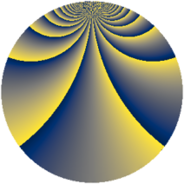# Properties

 Label 920.2.vLevel $920$ Weight $2$ Character orbit 920.v Rep. character $\chi_{920}(323,\cdot)$ Character field $\Q(\zeta_{4})$ Dimension $264$ Newform subspaces $1$ Sturm bound $288$ Trace bound $0$

# Related objects

## Defining parameters

 Level: $$N$$ $$=$$ $$920 = 2^{3} \cdot 5 \cdot 23$$ Weight: $$k$$ $$=$$ $$2$$ Character orbit: $$[\chi]$$ $$=$$ 920.v (of order $$4$$ and degree $$2$$) Character conductor: $$\operatorname{cond}(\chi)$$ $$=$$ $$40$$ Character field: $$\Q(i)$$ Newform subspaces: $$1$$ Sturm bound: $$288$$ Trace bound: $$0$$

## Dimensions

The following table gives the dimensions of various subspaces of $$M_{2}(920, [\chi])$$.

Total New Old
Modular forms 296 264 32
Cusp forms 280 264 16
Eisenstein series 16 0 16

## Trace form

 $$264q - 12q^{8} + O(q^{10})$$ $$264q - 12q^{8} + 16q^{10} - 16q^{12} - 16q^{16} + 8q^{17} - 28q^{18} - 16q^{22} - 8q^{25} - 48q^{26} - 12q^{28} - 24q^{30} + 40q^{32} - 48q^{35} + 32q^{36} + 12q^{38} + 16q^{40} + 40q^{42} - 64q^{43} - 28q^{48} - 40q^{50} + 16q^{52} - 40q^{56} - 20q^{58} - 100q^{60} - 60q^{62} - 8q^{65} - 72q^{66} + 48q^{67} + 72q^{68} - 60q^{70} + 80q^{72} - 40q^{73} + 112q^{75} + 48q^{76} + 28q^{78} - 60q^{80} - 264q^{81} + 44q^{82} + 56q^{86} - 68q^{88} + 64q^{91} + 16q^{96} - 40q^{97} + 16q^{98} + O(q^{100})$$

## Decomposition of $$S_{2}^{\mathrm{new}}(920, [\chi])$$ into newform subspaces

Label Dim. $$A$$ Field CM Traces $q$-expansion
$$a_2$$ $$a_3$$ $$a_5$$ $$a_7$$
920.2.v.a $$264$$ $$7.346$$ None $$0$$ $$0$$ $$0$$ $$0$$

## Decomposition of $$S_{2}^{\mathrm{old}}(920, [\chi])$$ into lower level spaces

$$S_{2}^{\mathrm{old}}(920, [\chi]) \cong$$ $$S_{2}^{\mathrm{new}}(40, [\chi])$$$$^{\oplus 2}$$